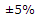# Electronics - Resistance and Power

Exercise : Resistance and Power - General Questions
51.
If a metallic conductor has a positive temperature coefficient of resistance, then:
as temperature increases, resistance decreases
as current increases, resistance decreases
as voltage increases, current increases
as temperature increases, resistance increases
Explanation:
No answer description is available. Let's discuss.

52.
What value of a1.3 kresistor as measured by a digital voltmeter would be considered within tolerance?
1234123513661367Explanation:
No answer description is available. Let's discuss.

53.
If a calculator display was "0.00263," what would this answer be in percent?
0.026%
0.26%
2.63%
26.3%
Explanation:
No answer description is available. Let's discuss.

54.
A 33 kresistor with a 20% tolerance checks out as ok with which of the following ohmmeter readings?
26400 ohms
24183 ohms
6600 ohms
39970 ohms